# Introduction Light

## Light of Class 9

Light is a form of electromagnetic energy that causes the sensation of vision.

## NATURE OF LIGHT

The study of light is called optics and is classified in the following ways: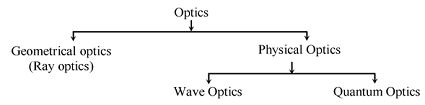### OPTICS

It is the branch of physics that deals with the study of light. It is mainly divided into three parts:

• Geometrical optics or optics: Geometrical optics, or ray optics, describes light propagation in terms of “rays”.
• Wave or physical optics: It is concerned with nature of light and deals with interference, diffraction and polarization. Wave motion is easily visualized in terms of water waves— such as those created on A quiet pond by a bobbing cork. The successive high points (crests) and low points (troughs) occur as a train of circular waves moving radially outward from the bobbing cork. Each of the circular waves represents a wave front. A wave front is defined here as a locus of points that connect identical wave displacements—that is, identical positions above or below the normal surface of the quiet pond.
• Quantum optics: It deals with the interaction of light with the atomic entities of matter such as Photo Electric effect, atomic excitation etc. Light propagating in avacuumhas its energy and momentum quantized according to an integer number of particles known as photons. Quantum optics studies the nature and effects of light as quantized photons.

PARTICLE THEORY OF LIGHT: Newton said that light travels in space with a great speed as a stream of very small particles called corpuscles. This theory failed to explain the interference of light as well as the diffraction of light. So a wave theory of light was accepted. Many known properties of light could be explained easily by a particle model.

For example, it was known that when light reflects from a smooth surface, the angle of incidence is equal to the angle of reflection.

This is also how an elastic, frictionless ball bounces from a smooth surface.

### WAVE THEORY OF LIGHT

According to the wave theory, light consists of electromagnetic waves. The light waves travel with a very high speed of 3 × 10 8 m/s in vacuum. The wavelength of visible light waves lies between 4 × 10 – 7 m to 8 × 10 – 7 m. The phenomenon of diffraction (bending of light around the corners of tiny objects) interference and polarization of light can only be explained if the light is considered to be of wave nature.

Around the same time, as Newton formulated his corpuscles' theory, his contemporary Huygen formulated the wave theory of light. The predominant model of wave propagation upto the end of the 19th century was modulations in some medium, therefore a medium called ether was hypothesized for waves of light to be produced due to vibrations of the particles of ether. The necessity of hypothesizing ether which is different than any material medium known to us, arises because light travels through empty space for example from sun and stars as well as dense objects like glass, diamond etc.

### MODERN QUANTUM THEORY OF LIGHT

Quantum theory tells us that both light and matter consist of tiny particles which have wave-like properties associated with them. Light is composed of particles called photons, and matteris composed of particles called electrons, protons, neutrons. It's only when the mass of a particle gets small enough that its wave like properties show up.

To help understand all this let's look at how light behaves as a wave and as a particle.

Wave-like Behavior of Light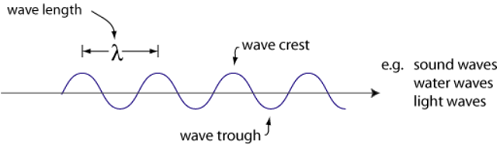When light falls on the surface of metals like Caesium, Potassium, etc., Electrons are thrown out. These electrons are called ‘photo-electrons’ and the phenomenon is called ‘photo-electric effect.’

This was explained by Einstein. According to Planck, light consisted of packets or quanta of energy called photons. Plank also studied this problem much earlier and constructed a mathematical model, according to which light consists of packets or quantas of energy. Einestein explained this later in 1905. He named a quantum of energy as ‘Photon’. The rest mass of photon is zero. Each quanta carries energy E= hv.

v → Planck’s constant = 6.6 × 10 -34 J-s.

v → frequency of light

Einstein’s photo-electric equation is h(v-v0) = 1/2 mv2 max

hνo = amount of energy spent in ejecting and electron out of metal surface.

V max = maximum velocity of the ejected electron.

Some phenomenon’s like interference of light, and diffraction of light are explained with the help of wave theory but wave theory failed to explain the photoelectric effect. It was explained with the help of quantum theory. So, light has dual nature.

(i) Particle nature (ii) Wave nature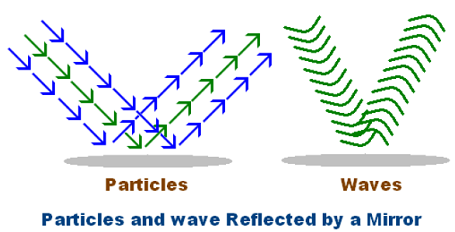### ELECTROMAGNETIC WAVES

Light, microwaves, x-rays, and TV and radio transmissions are all kinds of electromagnetic waves. They are all the same kind of wavy disturbance that repeats itself over a distance called the wavelength.

The waves which do not require a material medium for their propagation are known as electromagnetic waves. e.g. All light waves are electromagnetic waves.

### SOURCE OF LIGHT:

A body that emits light in all directions is said to be the source of light. The source can be a point source one or an extended one. The sources of light are of two types:

• Luminous source: Any object that by itself emits light is called as a luminous source. e.g. Sun and Stars (natural Luminous sources), Electric lamps, Candles and Lanterns (artificial luminous sources).
• Non-luminous source: Those objects which do not emit light but become visible when light from luminous objects falls on them and they reflect it back. They are called non-luminous. e.g. Moon, Planets (natural non-luminous sources), Wood, Table (artificial non-luminous sources).

### TYPES OF MEDIUM:

When light falls on a medium, it can be treated in three ways.

A medium is a substance through which light propagates or tries to do so. Based on this interaction mediums are classified into three categories.

• Transparent: The medium which allows most of the light to pass through it is called a transparent medium. e.g. Air, Water, Glass etc.
• Translucent: The medium which allows only a part of the light to pass through it is called a translucent medium. e.g. Paper, Ground glass etc.
• Opaque: The medium which does not allow any light to pass through it is called opaque medium. e.g. Wood, Bricks, Metals etc.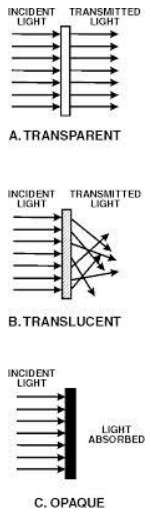### RAY:

A ray of light is the straight line path along which the light travels. It is represented by an arrow head on a straight line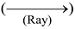. The direction of the arrow gives the direction of propagation of light.Imagining light as a ray makes it easy to describe, with great accuracy, three well-known phenomena: reflection, refraction and scattering. Let's take a second to discuss each one.

### BEAM OF LIGHT

A light beam or beam of light is a directional projection of light energy radiating from a light source.Sunlight forms a light beam(a sunbeam) when filtered through media such as clouds,foliage, or windows.

A group of parallel rays is called a beam of light. It is also called a pencil of light.

A pencil of light or a beam of light may be of three types.

• Convergent Pencil of light
• Divergent Pencil of light
• Parallel Pencil of light

### Convergent Pencil of light

Convergent Pencil is also known as convergent beam of light. In this case, as the beam of light progresses the rays converge to a point.

Or

A convergent Pencil is that in which rays of light propogate to meet at a particular point say O as shown in the figure.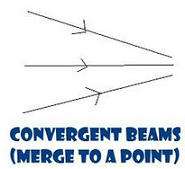Convergent Pencil of light

The diameter of the pencil goes on decreasing as the rays proceed forward.

Divergent Pencil of light

A divergent pencil is also known as a divergent beam of light. Here, as the beam of light progresses, the rays diverge from each other.

Or

A divergent pencil is that in which rays of light come out of a point source such that the diameter of the pencil goes on increasing as the rays proceed forward, as shown in the figure.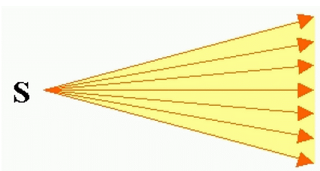Divergent Pencil of light

### Parallel Pencil of light

A parallel pencil is that in which all the rays move parallel to one another. The diameter of the pencil remains constant throughout as shown in figure 3.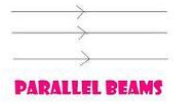Parallel Pencil of light

### BEHAVIOUR OF LIGHT AT THE INTERFACE OF TWO MEDIA:

When light traveling in one medium falls on the surface of a second medium the following three effects may occur:

(i) A part of the incident light is turned back into the first medium. This process is called the reflection of light.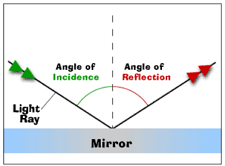(ii) A part of the incident light is transmitted into the second medium along a changed direction. This is called refraction of light.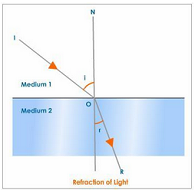(iii) The remaining third part of light energy is absorbed by the second medium. This is called absorption of light.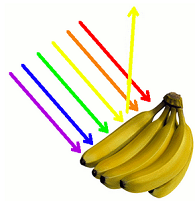We can understand all three behavior as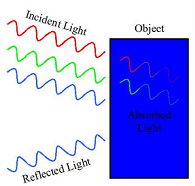To score More in your class 9 refer NCERT solutions for class 9.